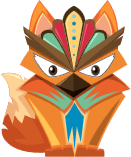Gerenimot# Numbers generator

40Hi, It's me Gerenimot!
Generate a number.

## Numbers and Numbers

Before we begin, we need to differentiate between a digit and a number. Numbers range from zeros to nine and we must not forget that zero is a digit. Numbers on the other hand are composed of one or more digits, so there is an infinite amount of numbers. Some specific numbers are represented by a symbol or a letter, Pi is the best example. We will see how numbers are represented in our digital information systems and how they are used.

### Numbers can take many forms in mathematics

Mathematics uses numbers in many forms to simplify understanding and writing certain equations. Putting a number in fractions can avoid writing a number with a decimal point that is too long, such as 1/3 which is equal to 0.333...333 to infinity.
Putting numbers in exponents as with scientific notation solves quite a few problems related to the size of numbers.

### The very large numbers, gogol and gogolplex

Names have been given to the largest numbers, these numbers cannot be interpreted by humans because they are so large. A gogol, pronounced google is equal to 1 plus 100 zeros behind it. It is an extremely large number because compared to the number of atoms in the universe which is 1 plus 80 zeros, it is much larger. The gogolplex is equal to 1 plus 1 gogol of zeros behind it. To understand so much it is big and difficult to apprehend that one could not write it on a sheet of paper. Indeed the number of zeros to write and higher than the number of atoms in the universe.

.

### Numbers and their numerical representations

In computing numbers are represented by bits, have the calls of binary numbers. A bit has only 2 possible positions 0 or 1.

### Different bases for counting

Computers use binary, humans use the decimal base but there are still other bases. The hexadecimal base is very important in computing it goes from 0 to 16 but from 10 we use letters:0,1,2,3,5,6,7,8,9,a,b,c,d,e,f. With this system 8 bits make 256 or in hexa FF.
A lesser known base is octodecimal based on the number 8.

## The draw and randomness

It should be noted that programs cannot do randomness, to use randomness they must create it through a sequence of mathematical operations or algorithm based most commonly on the passage of time or a seed. To draw a number at random from a computer, you need to call the random() function which will trigger an algorithm returning a result.

### Details of the random function

The random function is a pseudo-random function. It is mathematical method to create randomness, there are many different methods more or less greedy in resources and computing time. Random functions for computer security have complex methods to make the work of hackers more difficult. Another website for generate random numbers

.

### The use of randomness in video games.

We don't realize that random is used all around us. In Mario Kart, you grab a cube to get a power, at that moment, the random function chooses a number associated with a power.

## Gerenimot and the programming of randomness

Grace a node JS and Linux, Gerenimot uses the random function to give you a random number.